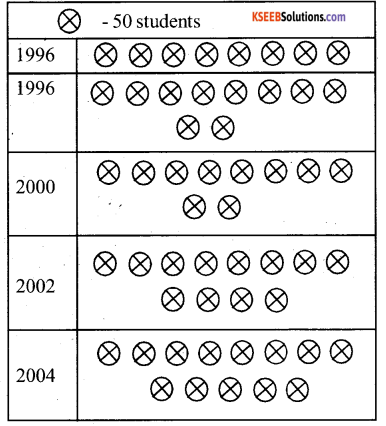# KSEEB Solutions for Class 6 Maths Chapter 9 Data Handling Ex 9.2

Students can Download Chapter 9 Data Handling Ex 9.2 Questions and Answers, Notes Pdf, KSEEB Solutions for Class 6 Maths helps you to revise the complete Karnataka State Board Syllabus and score more marks in your examinations.

## Karnataka State Syllabus Class 6 Maths Chapter 9 Data Handling Ex 9.2

Question 1.
Total number of animals in five villages are as follows: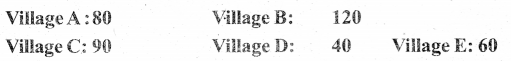Prepare a pictograph of these animals using one symbol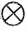to respect 10 animals and answer the following question:
a) How many symbols represent animals of village E ?
b) Which village has the maximum number of animals ?
c) Which village has more animals: village A or village C?
The pictograph for the given data can be drawn as follows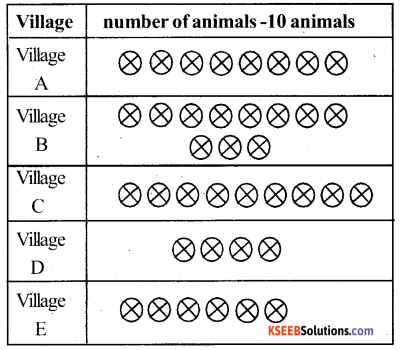a) 6 symbol will represent animals of village E as there were 60 animals in this village.
b) Village B has the maximum number of animals i.e., 120.
c) Village A and C have 80 and 90 animals in it clearly village C has more animals.Question 2.
Total number of students of a school in different years is shown in the following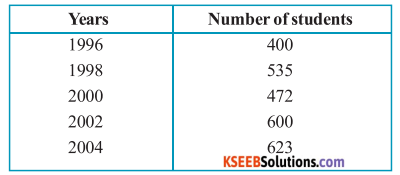A. Prepare a pictograph of students using one symbol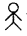to represent 100 students and answer the following questions:
Solution: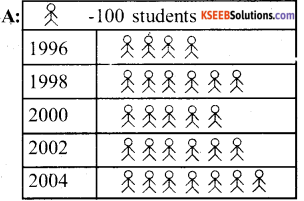a) How many symbols represent total number of students in the year 2002?
6 symbols represent the total number of students in the year 2002.

b) How many symbols represent total number of student for the year 1998?
5 Complete and 1 in complete symbols represent the total number of students in the year 1998.B. Prepare another pictograph of students using any other symbol each representing 50 students. Which pictograph do you find more informative?
Solution: